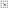## Journal directory listing - Volume 31-41 (1986-1996) - Volume 32 (1987)Directory Top

##### Some Remarks on Quasi-Injective Modules
Author: Cheng Mau-Hai

Abstract：

The purpose of this paper is to give some supplementary remarks of the previous work  by the author. The nota-tions and terminologies are referred to . We shall prove that for any quasi-injective R-module M, (i) if R is not a domain, then either TR(M)(TR(M))=M or TR(M)=(TR(M)) C (TR(M))=M; (ii) if R is a domain, then either TR(M)((TR(M))=M or TR(M)=(R(M)) is a proper direct summand of M.